Are you more of a visual learner? Check out our online video lectures and start your physics course now for free!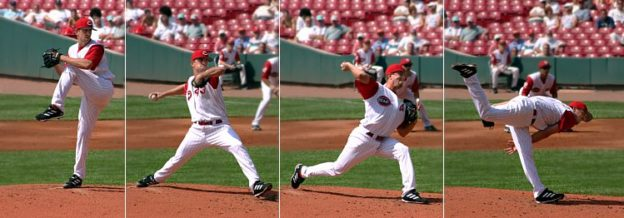Image: ”
Baseball pitching motion 2004.jpg. More details: Baseball pitching motion 2004.” License: CC BY-SA 3.0

Springs: Definition

Springs are elastic objects used to store mechanical energy in their coils owing to their ability to stretch and/or be compressed. They are usually referred to as coil springs. As a spring is compressed from its resting (non-mobile) position, it has the capability of exerting an opposing force in relation to the change in length it has undergone.

Restorative Force Capability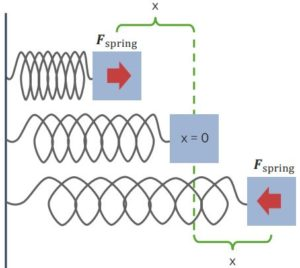“Springs Have Restorative Forces” Image created by Lecturio

Mechanical energy exists as kinetic and potential energies; the former is present when the spring is unstretched, while the latter is the energy in the system when the spring is stretched. When kinetic energy increases, the elastic potential energy correspondingly decreases.

The opposing force existing in the mechanical aspects of the spring is referred to as restorative force and is based on Hooke’s Law. This is the force required to restore the spring to its resting or equilibrium position.

The restorative force is dependent on the spring constant and displacement of the spring from its equilibrium position. The spring constant in turn is dependent on the strength of the spring and takes into consideration various physical properties such as the material of the spring and the diameter of the spring coil. The thicker the spring, the higher the spring constant. Conversely, thinner springs have lower spring constants.

The equation for restorative force can be represented as follows:

Fspring = – k x

k = spring constant

x = displacement of the spring from the equilibrium position

Fspring = restorative force

The equation requires a negative sign since the spring experiences a force pulling it back to its equilibrium position upon being stretched or compressed. This force acts in the opposite direction of the initial stretch or compression. In other words, the direction in which the spring stretches is opposite to the direction of the force it exerts.

The spring constant is always positive since only the magnitude is considered. It is represented as Newton per centimeter (N/cm) or Newton per millimeter (N/mm).

Springs have an elastic limit, which refers to the extent to which it can be stretched without altering its ability to return to its original length. As long as this limit is not exceeded, the spring displays elastic behavior; however, if exceeded, it portrays plastic behavior. Hooke’s law holds true only if the spring is within its elastic limit.

Oscillating Ability

Springs can oscillate; in other words, they can produce a repetitive variation in motion between their two extreme states, stretched and compressed, starting from a midpoint (the resting or equilibrium point).

When a spring is stretched and held in place, an object at the end of the spring has no velocity since it is not in motion. The force will act in a direction opposite to that of the stretch. This explains the negative sign in the equation representing Hooke’s Law. This force tries to restore the position of the spring to its equilibrium point. The displacement is denoted as ‘x,’ while the maximum displacement that the spring can undergo is represented as the maximum amplitude (A). When an object at the end of the spring is released, it will be pulled towards the equilibrium point with velocity ‘v.’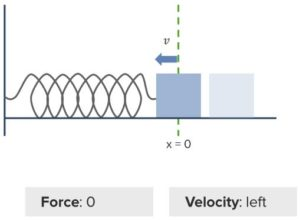“Spring Passes Equilibrium” Image created by Lecturio

At the equilibrium point, ‘x’ would equal 0, since the spring is passing through this point as it is being compressed to oppose the stretch. The spring continues to compress owing to its inherent velocity and passes equilibrium until it reaches the minimum amplitude at which the velocity is 0. At this point, the spring possesses a restorative force that acts in the opposite direction enabling it to return to equilibrium.

This cycle continues until the spring finally stops at the resting point. This back and forth motion comprising stretch and compress is termed an oscillation. Upon completion of several oscillations, the spring stops moving and returns to its resting point, where both ‘x’ and ‘v’ equal 0.

Spring Period and Frequency Calculations

Various aspects can be determined based on spring oscillations and their properties. The frequency ‘f’ indicates the number of cycles per second the spring undergoes, while the period ‘P’ denotes the time between oscillating motions. The two traits bear an inverse relationship and can be represented as P = 1/f.

Let us look at the frequency to determine the properties that affect this variable. The frequency is affected by the spring constant ‘k’ and mass of the spring ‘m.’

f = (1/2 π) √(k/m)

Thus, P = 2 π √(m/k).

Energy Conservation of a Spring

Let us now look at how kinetic and potential energies affect oscillations. When a spring is stretched and subsequently released, it moves through the equilibrium point. The initial energy prior to release equals the energy as the spring moves through the equilibrium point. This is the law of conservation of energy in a spring.

Thus,

Einitial = Efinal

Energy is a combination of kinetic energy ‘K’ and potential energy ‘U.’

So,

Kinitial + Uinitial = Kfinal + Ufinal

Before the spring is released after being stretched, the system can be considered to be at rest. At this point, kinetic energy does not come into play. Thus, Kinitial = 0. Similarly, since there is no potential energy at the equilibrium state, Ufinal = 0. This simplifies the equation to:

Uinitial = Kfinal

(1/2) k A2 = (1/2) m v2

This equation can be expanded, since potential energy is dependent on the stretched spring, whereas kinetic energy is dependent on the velocity of the spring motion.

Solving for velocity:

v = A

In other words, a stretched spring possesses only potential energy. Upon release, the restorative forces convert potential energy to kinetic energy and subsequently, at the equilibrium position, the spring possesses only kinetic energy. Conversely, when the spring compresses back to its minimal amplitude, its kinetic energy is completely converted to potential energy. This is similar to the potential- and kinetic-energy changes that occur due to the effect of gravity on an object.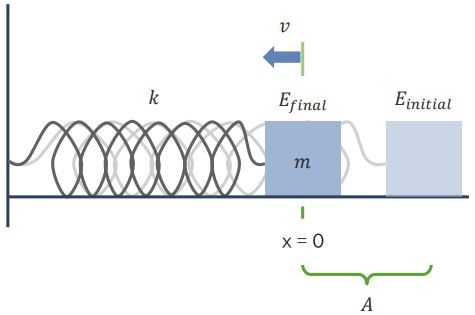“Energy Conservation in Springs” Image created by Lecturio

Applications

This principle is used in the manufacture of kitchen scales as well as devices that use springs, such as the suspension system in cars and retractable pens.

Pendulums: Definition

A pendulum is a weight suspended from a frictionless pivot enabling it to swing freely. Initially, before swinging, the pendulum rests straight downward at its resting or equilibrium position. Once it is displaced sideways, the swinging commences with oscillating back and forth motions. The pendulum swings in this manner since there is a restorative force to propel it to the equilibrium position.

Restorative Force Capability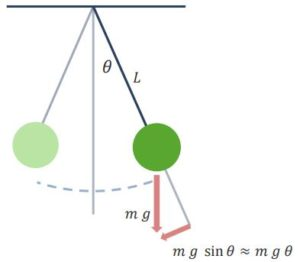“Pendulum” Image created by Lecturio

The restorative force of a pendulum drives it back to its equilibrium point after it has been displaced. The pendulum has a restorative force and oscillating pattern similar to that of a spring. A swinging pendulum passes through the resting position and continues to swing until it reaches a certain distance past the equilibrium point; it stops here momentarily and swings back in the opposite direction.

This oscillating motion continues until the pendulum eventually stops swinging.

It is assumed that there is no friction or air resistance in this model. Unlike a spring, the restorative force is dependent on gravity and the angle (ɵ) of motion from the midpoint, rather than the mass suspended from the pendulum. The pendulum is like a spring since the restorative forces for both depend on displacement.

F = m g sin ɵ

M = mass of the swinging object

g = gravity

ɵ = swing angle from the midpoint

However, for mathematical calculations, sin ɵ = ɵ for very small angles causing:

F = m g ɵ

Pendulum Period and Frequency Calculations

Various aspects can be determined based on the oscillations of a pendulum. The frequency ‘f’ indicates the number of oscillations of the pendulum per second, while the period ‘P’ denotes the time between oscillating motions. The two traits are inversely related and can be represented as P = 1/f

Let us look at the frequency to determine the properties that affect this variable. The frequency is affected by the gravitational energy ‘g’ and the length of the pendulum ‘L.’

f = 1/ [ 2π  √(L/g)]

Thus,

P = 2 π √(L/g)

It is to be noted that the mass on the pendulum does not affect its swing.

Energy Conservation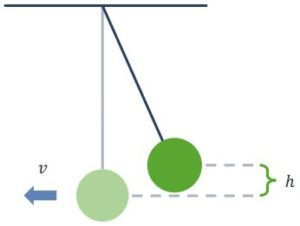“Energy Conservation of Pendulums” Image created by Lecturio

Pendulum oscillations are governed by kinetic and potential energies. When a pendulum is displaced and subsequently released, it moves through the equilibrium point.

The energy of the system before displacement equals the energy possessed by the pendulum as it moves through the equilibrium point. This is the law of conservation of energy in a pendulum.

Thus, Einitial = Efinal. Since there is a difference in the vertical location of the pendulum at its initial and final stops, the equation can be depicted as

Etop = Ebottom

Etop represents the energy prior to the release of the pendulum from its displaced position, while Ebottom represents the energy at the equilibrium point.

Energy is a combination of kinetic energy ‘K’ and potential energy ‘U.’

So,

Ktop + Utop = Kbottom + Ubottom

Before being released from its initial position, the kinetic energy of the pendulum, Ktop = 0. Additionally, at the equilibrium position, the potential energy of the system, Ubottom = 0. This simplifies the equation to:

Utop = Kbottom

mgh = (1/2) m v2

The equation can be expanded since potential energy is dependent on the displaced pendulum, whereas kinetic energy is dependent on the pendulum velocity.

Solving for velocity:

v =  √(2gh)

In other words, a pendulum before being displaced possesses only potential energy. Upon release, the restorative forces convert potential energy to kinetic energy, and subsequently, the pendulum at equilibrium possesses only kinetic energy. This cycle repeats as the pendulum swings back and forth. This phenomenon is similar to the changes in potential and kinetic energies that occur due to the effect of gravity on a free-falling object, or due to the compression/stretching oscillation of a spring.

Learn. Apply. Retain.
Your path to achieve medical excellence.
Study for medical school and boards with Lecturio.